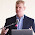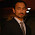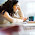Tuesday, December 9, 2014

Partitional clustering: number of clusters and performances a quantitative analysis

Abstract
Partitional clustering methods as k-medoid or k-means require an input parameter to specify the number of clusters to partition the data.
The complexity in time of such algorithms strictly depends on
• the number of clusters used to initialise the computation.
• the steps to update the centroids.
Whenever the similarity distance doesn't allow the determination of the centroid thru the analytical methods, the complexity in time tends to explode.
In the post I show an heuristic to minimise the complexity in time in non-Euclidean space.Number of computational steps for standard k-mean executed. Chart depicts  the steps by number of points $N$ in the range [1k,10k] and  by number of clusters in the range [5,$N/10$]. The cardinality of the clusters has been simulated assuming uniform distribution. The red line indicates the minimum number of steps.
Introduction
K-means or k-medoids are widely used because its implementation is very easy  and the complexity in time is low (it's linear over the iterations*#clusters#*#points).
Is the complexity linear  for whatever problem?

The problem
The problem arises when the objects to be clustered don't lie in a space for which the computation of the centroids can be done thru analytical methods.
In this context, the computational complexity to update the centroids becomes preponderant respect the other steps and the overall complexity is not any longer linear in time.

The complexity in case of non-Euclidean space
In a Euclidean space, the points of a cluster can be averaged.
In non-Euclidean spaces, there is no guarantee that points have an “average” (...some puritan of the measure theory might stuck up their nose on that) so we have to choose one of the members of the cluster as centroid.
As explained in the former post, for each cluster the computation of the centroid requires (in case of symmetric distance) $\binom{K_i}{2}$ computational steps.
The overall complexity (for each iteration) is the following:
$\sum_{i=1}^k \binom{z_i}{2}+k \cdot n$

A dataset having several points might require a number of computations simply not feasible; in that case it's essential the minimisation and optimisation of each param of the algorithm to reduce the complexity.
A numeric example will clarify the point.
Let's assume a data set of 10.000 elements, and let's assume that the compression rate of the clustering is 90% (meaning the number of clusters are 1.000).

• If the clusters cardinality is homogeneous, than the number of steps for the first iteration will be = 10.045.000.
• If the clusters cardinality is highly unbalanced, for instance in case that a cluster contains 95% of the points, than the number of steps is = 50.360.655.
As you can see... even if the data set is relatively small the computational effort is very high!

Determination of the #clusters that minimises the complexity in time

There are no many choices ... the only option is to determine the number of clusters that minimises the computational steps.

You might argue that the # clusters should be selected according to the distribution of the data set, but it's also true the following:
• In the real world you don't know a priori the exact number of clusters and all the heuristics to estimate them are quite often not really helpful in non-Euclidean space with several points. I prefer so, to have a sub-optimal clustering solution but with much faster answer :)
• clustering analysis is usually one of the many analysis that usually you perform to understand your data, therefore even if the clustering is not perfect, it doesn't hurt too much. Clustering it's just one piece of the entire puzzle!
The approach
If the clustering divides the data set in clusters having more or less the same size, the  solution is quite straightforward, we can consider the minimum of the function described above, thru the analysis of first derivate.
Assuming that each $z_i = \frac{n}{k}$ then the formula can be rewritten as:
$f(n,k)=\frac{n^2}{2 k}+k n-\frac{n}{2}$
The value of $k$ that minimises the first derivative of the such function is:
$\frac{\partial f(n,k) }{\partial k}=\frac{\partial}{\partial k} \frac{n^2}{2 k}+k n-\frac{n}{2} = n-\frac{n^2}{2 k^2}$.
And it takes value = 0 with $k= \frac{\sqrt{n}}{\sqrt{2}}$.

In all the other cases for which the clusters size is not homogeneous, then we can easily simulate it!Computations for a data set of 10k points.  The computations are estimated by number of clusters (cluster size ~ [2,1000]) and with unbalanced clusters sizes. The red line indicates the minimum.
As you can see the difference between  the computational steps to be executed in case of "best" configuration of #clusters and all the other possible configurations is quite impressive: it's almost 1*10^7 in worst case. And this is for each iteration!
I let you calculate how much time you can save working with the best configuration :).
Stay tuned!
c.

1.This is a very informative article about Data Mining. It is true that now a days data mining is playing a crucial role in the business growth or competition check, but we should done this job by experts only. Loginworks has expertise in providing the best data mining services on demand. Please check them at:

2.Internet Data Mining is a process that involves searching, collecting, filtering and analyzing the data from online sources. There are many Data Mining Company which providing the Data Mining Service

3.Thanks For this information. There are many Data Mining Companies which provides Data Mining Service, web data scraping and web data extraction services.

4.Thanks, these Data mining services information are really valuable. You have great search on it. Please write more on Benefits of Data Mining. Now you are subscribed.

5.Every one wants to get discounts on his online shopping purchase and this website is giving access to generate free amazon card via amazon gift card code generator that has the authority to provide up to 100\$ amazon card in a single go...!! just check this out and enjoy shopping online only at amazon gift card.

6.We at Coepd declared Data Science Internship Programs (Self sponsored) for professionals who want to have hands on experience. We are providing this program in alliance with IT Companies in COEPD Hyderabad premises. This program is dedicated to our unwavering participants predominantly acknowledging and appreciating the fact that they are on the path of making a career in Data Science discipline. This internship is designed to ensure that in addition to gaining the requisite theoretical knowledge, the readers gain sufficient hands-on practice and practical know-how to master the nitty-gritty of the Data Science profession. More than a training institute, COEPD today stands differentiated as a mission to help you "Build your dream career" - COEPD way.

http://www.coepd.com/AnalyticsInternship.html

7.COEPD LLC- Center of Excellence for Professional Development is the most trusted online training platform to global participants. We are primarily a community of Business Analysts who have taken the initiative to facilitate professionals of IT or Non IT background with the finest quality training. Our trainings are delivered through interactive mode with illustrative scenarios, activities and case studies to help learners start a successful career. We impart knowledge keeping in view of the challenging situations individuals will face in the real time, so that they can handle their job deliverables with at most confidence.

8.9.